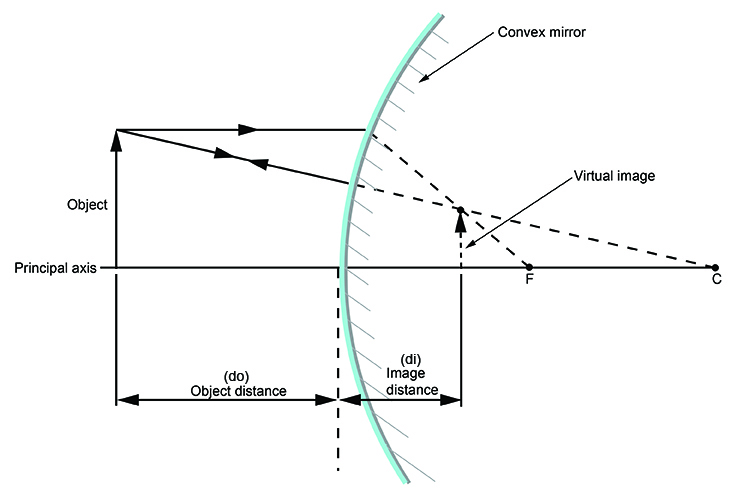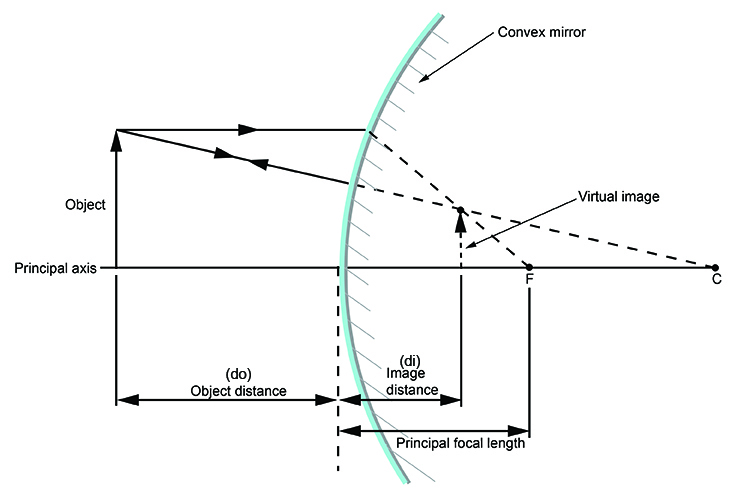# Object distance and image distance – Convex mirrors

The object distance is the distance from the object to the mirror. This is denoted by the symbol do.

Image distance (sometimes confused with the focal length) is the distance from the virtual image to the mirror. This is denoted by the symbol di.With the principal focal length added this would look like: